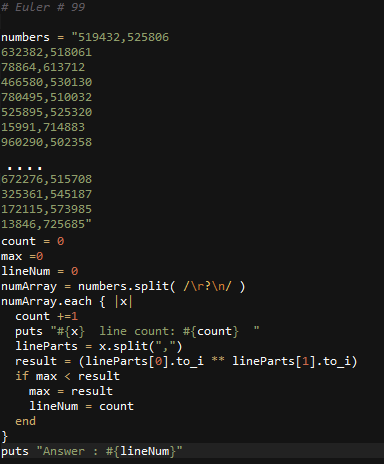# Ruby: Project Euler Problem # 99

http://projecteuler.net/problem=99

Largest exponential

Comparing two numbers written in index form like 211 and 37 is not difficult, as any calculator would confirm that 211 = 204837 = 2187.

However, confirming that 632382518061519432525806 would be much more difficult, as both numbers contain over three million digits.

Using base_exp.txt , a  text file containing one thousand lines with a base/exponent pair on each line, determine which line number has the greatest numerical value.